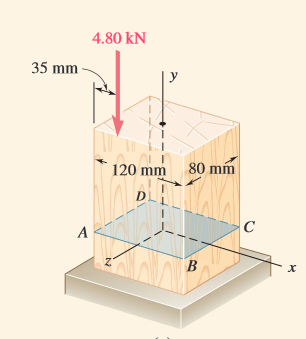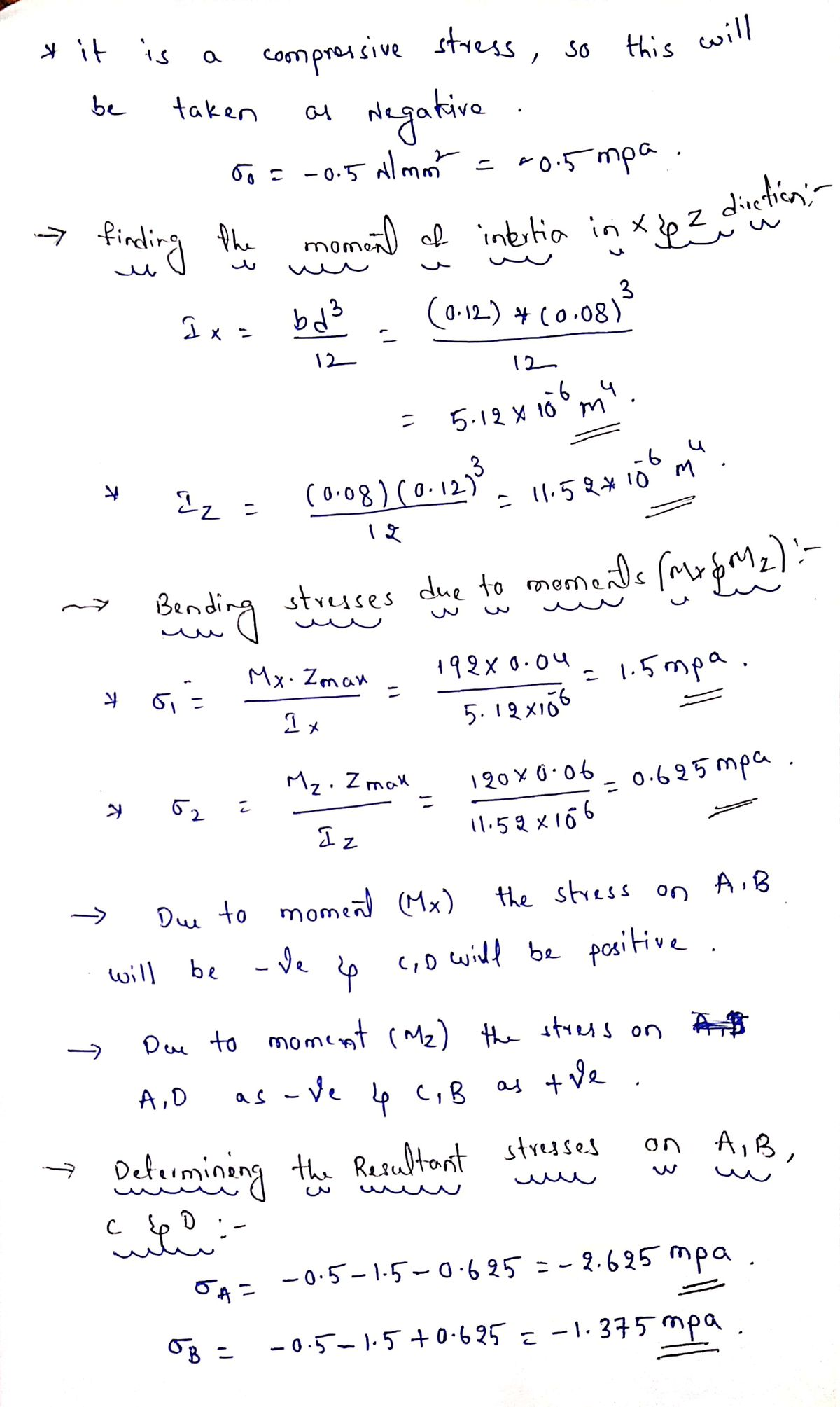# A vertical 4.80-kN load is applied as shown on a wooden post of rectangular cross section, 80 by 120 mm (Fig. 11.40a). (a) Determine the stress at points A, B, C, and D. (b) Locate the neutral axis of the cross section.

Question-AnswerCategory: Engineering MechanicsA vertical 4.80-kN load is applied as shown on a wooden post of rectangular cross section, 80 by 120 mm (Fig. 11.40a). (a) Determine the stress at points A, B, C, and D. (b) Locate the neutral axis of the cross section.

### A vertical 4.80-kN load is applied as shown on a wooden post of rectangular cross section, 80 by 120 mm (Fig. 11.40a). (a) Determine the stress at points A, B, C, and D. (b) Locate the neutral axis of the cross section.